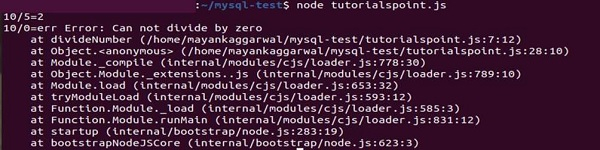# NodeJS - Exception Handling in Synchronous Code

An exception is a type of an event that occurs while executing or running a program that stops the normal flow of the program and returns to the system. When an exception occurs the method creates an object and gives it to the runtime system. This creating an exception and giving it to the runtime system is known as throwing an Exception.

We need to handle these Exceptions to handle any use case and prevent the system from crashing or performing an unprecedented set of instructions. If we donot handle or throw exceptions, the program may perform strangely.

## Exception handling in Synchronous Program

Here, we will learn how to handle exceptions in a synchronous flow of program. A synchronous program flow is where we return a response after completion when a request is received.

## Example

// Define a divide program as a syncrhonous function
var divideNumber = function(x, y) {
// if error condition?
if ( y === 0 ) {
// Will "throw" an error safely by returning it
// If we donot throw an error here, it will throw an exception
return new Error("Can not divide by zero")
} else {
// No error occurred here, continue on
return x/y
}
}

// Divide 10 by 5
var result = divideNumber(10, 5)
// Checking if an Error occurs ?
if ( result instanceof Error ) {
// Handling the error if it occurs...
console.log("10/5=err", result)
}
else {
// Returning the result if no error occurs
console.log("10/5="+result)
}

// Divide 10 by 0
result = divideNumber(10, 0)
// Checking if an Error occurs ?
if ( result instanceof Error ) {
// Handling the error if it occurs...
console.log("10/0=err", result)
}
else {
// Returning the result if no error occurs
console.log("10/0="+result)
}

## Output